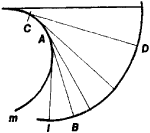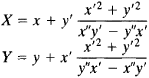# Evolute and Involute

The following article is from The Great Soviet Encyclopedia (1979). It might be outdated or ideologically biased.

## Evolute and Involute

concepts of differential geometry. The locus m of the centers of curvature of a plane curve l is called the evolute of l, and l is said to be an involute of m (see Figure 1).Figure 1

An involute l of a curve m is the curve described by the end B of the thread AB as it is unwound from m or wound on m. This construction of the involute makes clear the following properties of the evolute and involute: (1) The tangent CD at an arbitrary point C on the evolute is normal to the involute at the corresponding point D; consequently, an involute is an orthogonal trajectory of the tangents to the evolute. (2) Any orthogonal trajectory of the tangents to a curve m is an involute of m; m therefore has infinitely many involutes. (3) The difference between the radii of curvature AB and CD at the points B and D of an involute is equal to the length of the arc AC of the evolute. (4) The evolute is the envelope of the family of normals of an involute.

If a curve l is defined by the parametric equations x = x(t) and y = y(t), then the parametric equations of its evolute areAn involute of a space curve is an orthogonal trajectory of the tangents of the curve.

### REFERENCE

Rashevskii, P. K. Kurs differentsial’noi geometrii, 4th ed. Moscow, 1956.
The Great Soviet Encyclopedia, 3rd Edition (1970-1979). © 2010 The Gale Group, Inc. All rights reserved.
Site: Follow: Share:
Open / Close